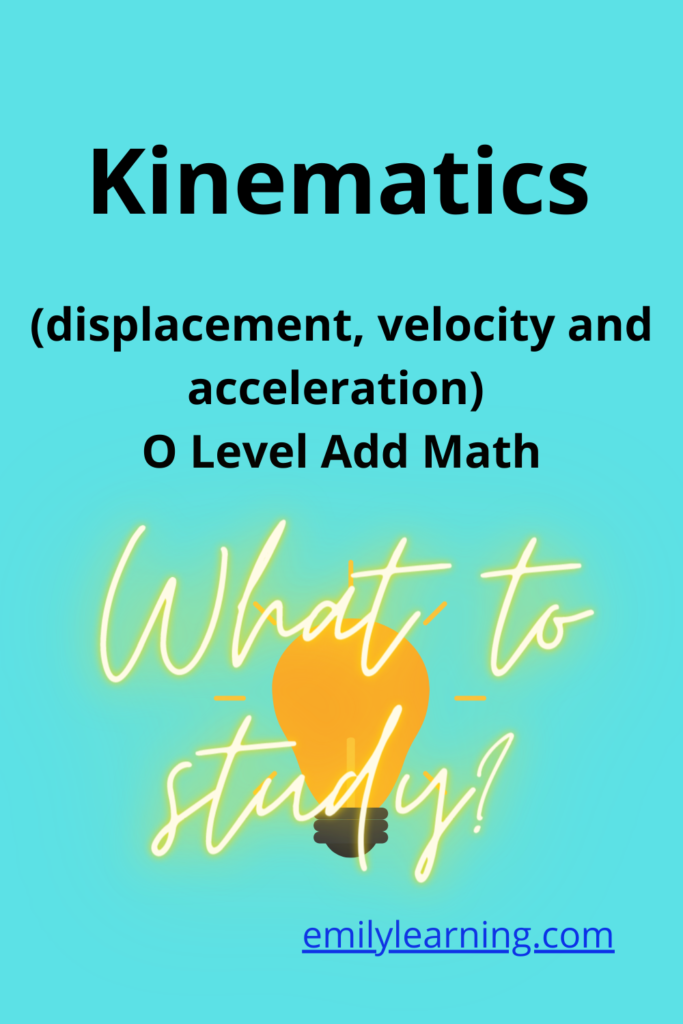# What you need to know for kinematics (displacement, velocity and acceleration) as tested in O Level Add MathApplication of integration and differentiation to kinematics is a chapter most schools cover after they completed all the chapters on integration and differentiation. Usually, one question from this topic comes out in the O Level each year.

To learn this chapter in detail, together with all other topics tested in O Level Additional Mathematics, do check out my online on-demand course on O Level Additional Mathematics.

So, what do you need to know for this chapter on kinematics?

Well, if you also take physics at O Level, then you must be familiar with displacement, velocity and acceleration. In this chapter in Additional mathematics, we write displacement (s), velocity (v) and/or acceleration (a) in Mathematical form and use integration and differentiation to find expressions for unknown terms. In short, you are given an expression of s, v or a in the exam, and are expected to find the others (e.g. s, v, or a that has not been given).

So these are what you need to know for this chapter:

• For a particle moving in a straight line, form expressions of s, v,  a using differentiation or integration.
• Solve problem sums involving displacement, velocity and acceleration of a particle moving in a straight line

## Want to learn O Level Additional Math on-demand?

Check out our on-demand O Level Additional Math course here.

error: Content is protected !!